# Power Meter Tutorials - Calibration

Yokogawa hopes that its Power meters contribute to a world with cleaner and more efficient energy use. The below tutorial intends to help with power measurement.

Calibration

1. Traceability of wide-bandwidth power meter
2. Calibration method for the working standard
3. Calibration at PF=0, Capacitor method
4. Calibration at PF=1

### Traceability of wide-bandwidth power meter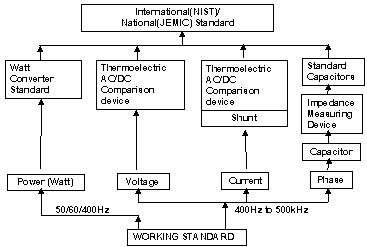### Calibration method for the working standard

1. Voltage calibration
2. Current calibration
3. Phase angle measurement of the capacitor
• Preparing some capacitors each frequency (50/ 60/ 400/ 1k/ 2k/ 5k / 10k/ 20k/ 50k/ 100k/ 200k/ 500kHz)
• Measuring admittance (Conductance-G and susceptance-X) of the capacitor using precision LCR meter
• Calculating the phase angle of the capacitor
1. Power calibration at PF=0
(It measures Internal phase difference between voltage and current input circuit.)
2. Power calibration at PF=1

### Power calibration at PF=0

Calibration Method for working standard at PF=0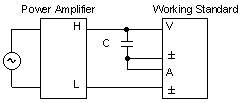Capacitor's Equivalent Circuit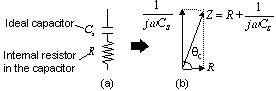Consumption power of the resistor in the capacitor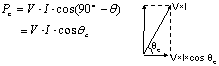Working standard displays power value (expectation)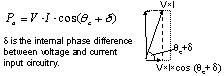Compensate of the input impedance of the voltage input circuit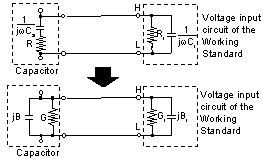Phase difference (_t) including the influence of input impedance of the voltage input circuit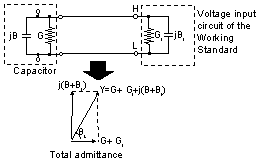Consumption power of the total admittance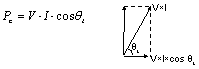Working standard displays power value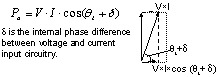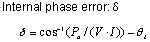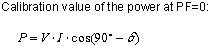### Power calibration at PF=1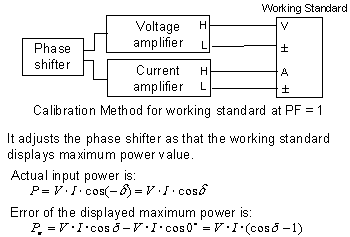### Related Products & Solutions

#### WT2010/WT2030 Digital Power Meters

The WT2000 digital powermeter series has been designed with emphasis on basic performance (bandwidth, accuracy, response speed, and noise immunity) from the viewpoint of measurement of electrical quantities. The broad range of functions of these power analyzers enable them to be used in various fields of applications.

Top